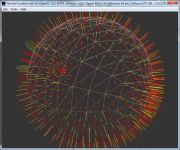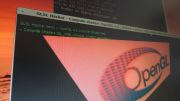Home Utilities Tutorials 3D Demos Graphics Cards Forums About

 GeeXLab
Current version: 0.29.17
>GeeXLab homepage

 FurMark
Current version: 1.21.1
>FurMark homepage

 GPU Caps Viewer
Current version: 1.44.2.1
>GPU Caps Viewer homepage

 GPU Shark
Current version: 0.16.1.0
>GPU Shark homepage

 Blogs
>JeGX's HackLab

 Geeks3D's Articles
>GPU Memory Speed Demystified

>How to Get your Multi-core CPU Busy at 100%

>How To Make a VGA Dummy Plug

>Night Vision Post Processing Filter

 PhysX FluidMark
Current version: 1.5.4
>FluidMark homepage

 TessMark
Current version: 0.3.0
>TessMark homepage

Current version: 0.3.0

 Demoniak3D
Current Version: 1.23.0
>Demoniak3D
>Libraries and Plugins
>Demos
>Codes Samples

3D Graphics Search Engine:Bump Mapping using GLSL By Jérôme 'JeGX' GUINOTjegx [at] ozone3d [dot] net Initial draft: November 4, 2005 Update: November 18, 2005 Update: March 8, 2006 Update: December 30, 2006 [ Index ]»Next Page2 - Lighting EquationsThe final color of the pixel displayed on the screen is given by the following equation:`If = Ia + Id + Is`where If is the intensity of the pixel final color, Ia is the intensity of the ambient color, Id is the intensity of the diffuse color and Is that of the specular color. For more explanations on these various components, please refer to the Lighting & Materials tutorial (soon available). Ia, Id and Is are all four-dimensional RGBA vectors. The Ia term is the ambient component. Ia is the result of the multiplication between the ambient component of the light and that of the material which composes the surface of the 3d object:`Ia = Al * Am`where Al is the ambient component of the light and Am that of the material. Ia is generally a constant RGBA vector, and this value is the same one independently from the pixel. We will see in another tutorial a more advanced expression of this ambient term with the technique known as the Ambient Occlusion Lighting. The Id term expresses the final diffuse component. This component is given by the following equation:`Id = Dl * Dm * LambertTerm`where Dl is the diffuse component of the light and Dm that of the material. The LambertTerm factor is the keystone of the lighting equations. It is indeed the value of this factor which will make it possible to create the self shadow of a 3d object (self-shadowing). This Lambert coefficient is calculated with the following dot product:`LambertTerm = max( N dot L, 0.0)`where N is the normal vector to the considered pixel and L the light vector at the same pixel. This simple relation but so fundamental, tells us that the value of the Lambert coefficient will be maximum (1.0) if the angle between the two vectors (L and N) equals zero, i.e. if the pixel is directly in front of the light. For all the other cases, the Lambert coefficient will vary between 0.0 and 1.0 what will generate the self shadow. The max() function is just there to prevent us from having a negative value for the Lambert term. Update: March 8, 2006: The Is term expresses the final specular component. This component is obtained by:`Is = Sm x Sl x pow( max(R dot E, 0.0), f )`The Is term is from far the most complicated to calculate but it is responsible of these famous specular reflections on the surface of the objects. Sl is the specular component of the light and Sm that of the material. E is the view vector or camera vector and R is the light L reflected vector in relation to the normal N. R is obtained with:`R = reflect(-L, N)`where N is the normal vector to the pixel considered, L the light vector and reflect() a function (available in GLSL) which makes it possible to calculate the reflexion vector of L in relation to N. The pow() function is the power function which makes it possible to raise a number N to the power of p: pow(n, p). f is the specular exponential factor (the famous shininess in OpenGL) which represents the hardness and the precision of the specular reflection. These small explanations show us the importance of the N normal vector. In traditional rendering process, the N vector at the level of a pixel results from the interpolation of the three normal vectors of the three vertices which form the current face of the 3D object. In this case the variations of the N vector are very small on the current face. The bump mapping technique precisely consists in giving more life and sparkling to this poor N vector by drawing for each pixel a normal vector from a normal-map. For more details on the normal map, please refer to this tutorial: Normal Maps. The textures used for this tutorial are the same ones as those of the normal-maps tutorial.[ Index ]»Next Page

 GeeXLab demosGLSL - Mesh exploderPhysX 3 cloth demoNormal visualizer with GS